# pylops.signalprocessing.FFT¶

pylops.signalprocessing.FFT(dims, dir=0, nfft=None, sampling=1.0, norm='ortho', real=False, fftshift=None, ifftshift_before=None, fftshift_after=False, engine='numpy', dtype='complex128', **kwargs_fftw)[source]

One dimensional Fast-Fourier Transform.

Apply Fast-Fourier Transform (FFT) along a specific direction dir of a multi-dimensional array of size dim.

Using the default NumPy engine, the FFT operator is an overload to either the NumPy numpy.fft.fft (or numpy.fft.rfft for real models) in forward mode, and to numpy.fft.ifft (or numpy.fft.irfft for real models) in adjoint mode, or their CuPy equivalents. When engine='fftw' is chosen, the pyfftw.FFTW class is used instead. Alternatively, when the SciPy engine is chosen, the overloads are of scipy.fft.fft (or scipy.fft.rfft for real models) in forward mode, and to scipy.fft.ifft (or scipy.fft.irfft for real models) in adjoint mode.

When using real=True, the result of the forward is also multiplied by $$\sqrt{2}$$ for all frequency bins except zero and Nyquist, and the input of the adjoint is multiplied by $$1 / \sqrt{2}$$ for the same frequencies.

For a real valued input signal, it is advised to use the flag real=True as it stores the values of the Fourier transform at positive frequencies only as values at negative frequencies are simply their complex conjugates.

Parameters: dims : tuple Number of samples for each dimension dir : int, optional Direction along which FFT is applied. nfft : int, optional Number of samples in Fourier Transform (same as input if nfft=None) sampling : float, optional Sampling step dt. norm : {“ortho”, “none”, “1/n”}, optional New in version 1.17.0. “ortho”: Scales forward and adjoint FFT transforms with $$1/\sqrt{N_F}$$, where $$N_F$$ is the number of samples in the Fourier domain given by nfft. “none”: Does not scale the forward or the adjoint FFT transforms. “1/n”: Scales both the forward and adjoint FFT transforms by $$1/N_F$$. Note For “none” and “1/n”, the operator is not unitary, that is, the adjoint is not the inverse. To invert the operator, simply use Op \ y. real : bool, optional Model to which fft is applied has real numbers (True) or not (False). Used to enforce that the output of adjoint of a real model is real. fftshift : bool, optional Deprecated since version 1.17.0: Use ifftshift_before. ifftshift_before : bool, optional New in version 1.17.0. Apply ifftshift (True) or not (False) to model vector (before FFT). Consider using this option when the model vector’s respective axis is symmetric with respect to the zero value sample. This will shift the zero value sample to coincide with the zero index sample. With such an arrangement, FFT will not introduce a sample-dependent phase-shift when compared to the continuous Fourier Transform. Defaults to not applying ifftshift. fftshift_after : bool, optional New in version 1.17.0. Apply fftshift (True) or not (False) to data vector (after FFT). Consider using this option when you require frequencies to be arranged naturally, from negative to positive. When not applying fftshift after FFT, frequencies are arranged from zero to largest positive, and then from negative Nyquist to the frequency bin before zero. engine : str, optional Engine used for fft computation (numpy, fftw, or scipy). Choose numpy when working with cupy arrays. Note Since version 1.17.0, accepts “scipy”. dtype : str, optional Type of elements in input array. Note that the dtype of the operator is the corresponding complex type even when a real type is provided. In addition, note that neither the NumPy nor the FFTW backends supports returning dtype different than complex128. As such, when using either backend, arrays will be force-casted to types corresponding to the supplied dtype. The SciPy backend supports all precisions natively. Under all backends, when a real dtype is supplied, a real result will be enforced on the result of the rmatvec and the input of the matvec. **kwargs_fftw Arbitrary keyword arguments for pyfftw.FTTW ValueError If dims is provided and dir is bigger than len(dims). If norm is not one of “ortho”, “none”, or “1/n”. NotImplementedError If engine is neither numpy, fftw, nor scipy.

FFT2D
Two-dimensional FFT
FFTND
N-dimensional FFT

Notes

The FFT operator (using norm="ortho") applies the forward Fourier transform to a signal $$d(t)$$ in forward mode:

$D(f) = \mathscr{F} (d) = \frac{1}{\sqrt{N_F}} \int\limits_{-\infty}^\infty d(t) e^{-j2\pi ft} \,\mathrm{d}t$

Similarly, the inverse Fourier transform is applied to the Fourier spectrum $$D(f)$$ in adjoint mode:

$d(t) = \mathscr{F}^{-1} (D) = \frac{1}{\sqrt{N_F}} \int\limits_{-\infty}^\infty D(f) e^{j2\pi ft} \,\mathrm{d}f$

where $$N_F$$ is the number of samples in the Fourier domain nfft. Both operators are effectively discretized and solved by a fast iterative algorithm known as Fast Fourier Transform. Note that the FFT operator (using norm="ortho") is a special operator in that the adjoint is also the inverse of the forward mode. For other norms, this does not hold (see norm help). However, for any norm, the Fourier transform is Hermitian for real input signals.

Attributes: dims_fft : tuple Shape of the array after the forward, but before linearization. For example, y_reshaped = (Op * x.ravel()).reshape(Op.dims_fft). f : numpy.ndarray Discrete Fourier Transform sample frequencies real : bool When True, uses rfft/irfft rdtype : bool Expected input type to the forward cdtype : bool Output type of the forward. Complex equivalent to rdtype. shape : tuple Operator shape clinear : bool New in version 1.17.0. Operator is complex-linear. Is false when either real=True or when dtype is not a complex type. explicit : bool Operator contains a matrix that can be solved explicitly (True) or not (False)

## Examples using pylops.signalprocessing.FFT¶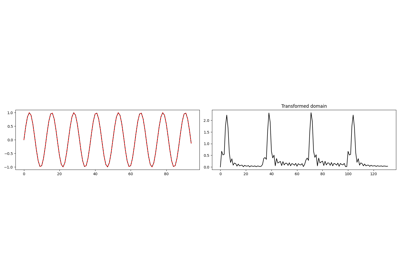1D, 2D and 3D Sliding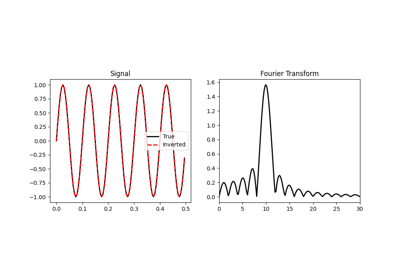Fourier Transform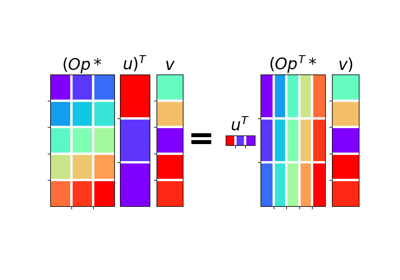02. The Dot-Test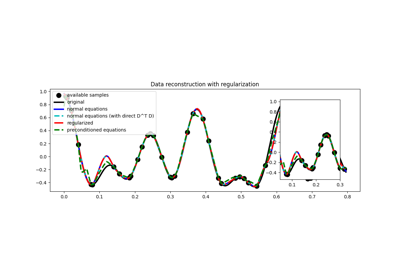03. Solvers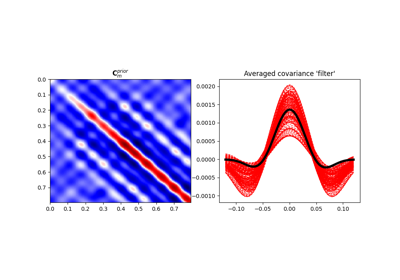04. Bayesian Inversion